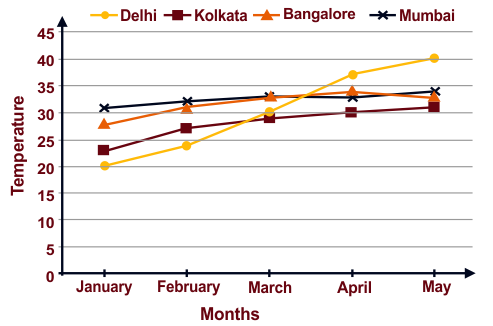# Unified Council - UIMO PDF Sample Papers for Class 6

Class 6 sample paper & practice questions for Unified International Mathematics Olympiad (UIMO) level 1 are given below. Syllabus for level 1 is also mentioned for these exams. You can refer these sample paper & quiz for preparing for the UIMO exam.#### Resources:

##### Sample Questions from Olympiad Success:
 Q.1 Q.2 Q.3 Q.4 Q.5 Q.6 Q.7 Q.8 Q.9 Q.10
 Q.1 The ratio of sugar and salt in a particular solution is 3:5. If 12 cubes of sugar are added, then find the cubes of salt added to the solution. a) 22 b) 21 c) 20 d) 19
 Q.2 Ram’s bank balance is Rs. 500 more than 3 times his friend’s bank balance. If his friend’s bank balance is ‘ y ‘. Then what is Ram’s bank balance? a) y + 500 b) 3y - 500 c) 3y d) 3y + 500
 Q.3 If each match box contains 50 matchsticks, the number of matchsticks required to fill in such boxes is: a) 50 + n b) 50n c) 50/n d) 50 - n
 Q.4 Here is the average monthly temperature of the following cities. With respect to this, answer question. Which city is the hottest in May?a) Kolkata b) Bangalore c) Delhi d) Mumbai
 Q.5 Ram was playing with 2 dice. He threw it 10 times and following were the results (3, 5), (1, 6), (2, 2), (3, 6), (6, 3), (6, 1), (2, 5), (1, 3), (2, 1), (6, 6) Which number appears the most number of times? a) 1 b) 2 c) 3 d) 6
 Q.6 Which of the following is a prime number? a) 93 b) 81 c) 33 d) 97
 Q.7 Form the equations: Sum of 2 times x and 3 times y is 1. a) 2x = 3y + 1 b) 3x + 2y = 1 c) 2x + 3y = 1 d) 3x = 2y + 1
 Q.8 Identify the property used in the following: 2 x 13 + 8 x 13 = ( 2 + 8 ) x 13. a) Distributive b) Commutative c) Closer d) Associative
 Q.9 If 2x + 3 = 5, which of the following is the value of ‘x’? a) -4 b) 4 c) -1 d) 1
 Q.10 3 X 10000 + 7 X 1000 + 9 X 100 + 0 X 10 + 4 is the same as: a) 37914 b) 37940 c) 37904 d) 379409Sample PDF of Unified Council - Unified International Mathematics Olympiad (UIMO) PDF Sample Papers for Class 6:

 Q.1 )c Q.2 )d Q.3 )b Q.4 )c Q.5 )d Q.6 )d Q.7 )c Q.8 )a Q.9 )d Q.10 )c

Q.1 : c | Q.2 : d | Q.3 : b | Q.4 : c | Q.5 : d | Q.6 : d | Q.7 : c | Q.8 : a | Q.9 : d | Q.10 : c